Download Presentation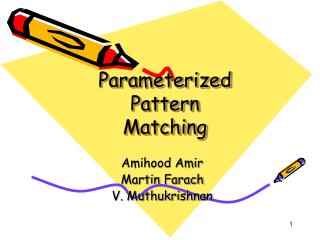Parameterized Pattern Matching

# Parameterized Pattern Matching - PowerPoint PPT Presentation

Parameterized Pattern Matching. Amihood Amir Martin Farach V. Muthukrishnan. Parameterized Matching. Input: two strings s and t |s|=|t|, over alphabets ∑ s and ∑ t . s parameterize matches t: if bijection : ∑ s ∑ t , such that (s) = t. Example:. a. a. b. b. b.I am the owner, or an agent authorized to act on behalf of the owner, of the copyrighted work described.
Download Presentation## Parameterized Pattern Matching

An Image/Link below is provided (as is) to download presentation

Download Policy: Content on the Website is provided to you AS IS for your information and personal use and may not be sold / licensed / shared on other websites without getting consent from its author.While downloading, if for some reason you are not able to download a presentation, the publisher may have deleted the file from their server.

- - - - - - - - - - - - - - - - - - - - - - - - - - E N D - - - - - - - - - - - - - - - - - - - - - - - - - -
Presentation Transcript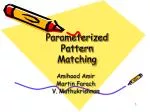### Parameterized Pattern Matching

Amihood Amir

Martin Farach

V. Muthukrishnan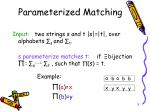Parameterized Matching

Input: two strings s and t |s|=|t|, over alphabets ∑s and ∑t.

s parameterize matches t: if bijection : ∑s ∑t , such that (s) = t.

Example:

a

a

b

b

b

(a)=x

x

x

y

y

y

(b)=y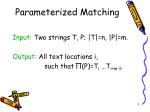Parameterized Matching

Input: Two strings T, P; |T|=n, |P|=m.

Output: All text locations i,

such that (P)=Ti …Ti+m-1.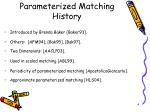Parameterized Matching History
• Introduced by Brenda Baker [Baker93].
• Others: [AFM94], [Bak95], [Bak97].
• Two Dimensions: [AACLP03].
• Used in scaled matching [ABL99].
• Periodicity of parameterized matching [ApostolicoGiancarlo].
• Approximate parameterized matching [HLS04].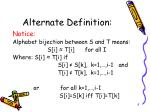Alternate Definition:

Notice:

Alphabet bijection between S and T means:

S[i] ≈ T[i] for all I

Where: S[i] ≈ T[i] if

S[i] ≠ S[k], k=1,…,i-1 and

T[i] ≠ T[k], k=1,…,i-1

or for all k=1,…,i-1

S[i]=S[k] iff T[i]=T[k]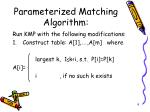Parameterized Matching Algorithm:

Run KMP with the following modifications:

• Construct table: A,…,A[m] where

largest k, 1≤k<i, s.t. P[i]=P[k]

A[i]=

i , if no such k exists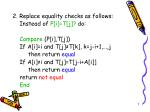2. Replace equality checks as follows:

Instead of P[i]=T[j]? do:

Compare (P[i],T[j])

If A[i]=i and T[j]≠T[k], k=j-i+1,…,j

then return equal

If A[i]≠i and T[j]=T[j-i+A[i]]

then return equal

return not equal

End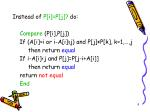Instead of P[i]=P[j]? do:

Compare (P[i],P[j])

If (A[i]=i or i-A[i]≥j) and P[j]≠P[k], k=1,…,j

then return equal

If i-A[i]<j and P[j]=P[j-i+A[i]]

then return equal

return not equal

End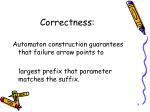Correctness:

Automaton construction guarantees that failure arrow points to

largest prefix that parameter matches the suffix.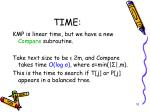TIME:

KMP is linear time, but we have a new Compare subroutine.

Take text size to be ≤ 2m, and Compare takes time O(log σ), where σ=min(|Σ|,m).

This is the time to search if T[j] or P[j] appears in a balanced tree.TIME:

Automaton Construction: O(m log σ) .

Text Scanning: O(n log σ) .

Can we do better?Alphabet Σ={1,…,n}

Can be done in linear time.

How?

Construct array:

1: list of indices of symbol 1

2: list of indices of symbol 2

.

.

m: list of indices of symbol m.To check if T[j]≠T[k], k=j-i+1,…,j

Assume the symbol in T[j] is a.

Check if

previous index to j in a’s list < j-i+1LOWER BOUNDS

What about general alphabets?

Element distincness Problem (EDP)

Input: Array A,…,A[n] of natural numbers.

Decide: If all elements of A are distinct (i.e. no i≠j where A[i]=a[j])TIME FOR EDP:

In comparison model:

General alphabets:Ω(n log n)

Alphabet Σ={1,…,n}:linear time.

(construct array of indices)Linear Reduction

Claim: EDP is linearly reducible to Parameterized Matching.

Proof: Let A,…,A[n] be an array of numbers.

In linear time, check if A is unique.

If so, construct S=A,A,…,A[n],ALinear Reduction (cont.)

A ≈ S iff all elements of A are distinct.

trivial

By induction on the prefixes of A.

A is unique – we checked.

Assume A,…,A[k] are distinct.

In particular, A[k] is unique.Linear Reduction (cont.)

But A[k] was parameter-matched to S[k], so S[k] only appears once in S.

But S[k]=A[k+1].

This means that A[k+1] is unique.